Polar representation

Chapter 5 Class 11 Complex Numbers
Concept wise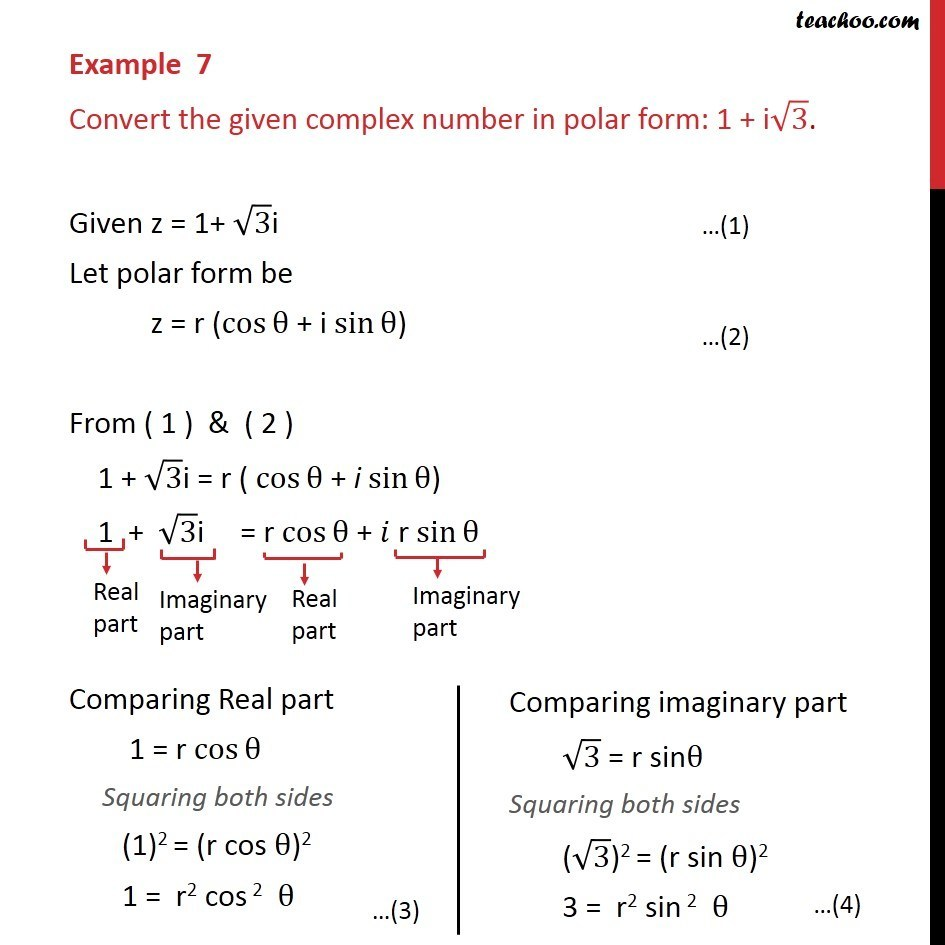a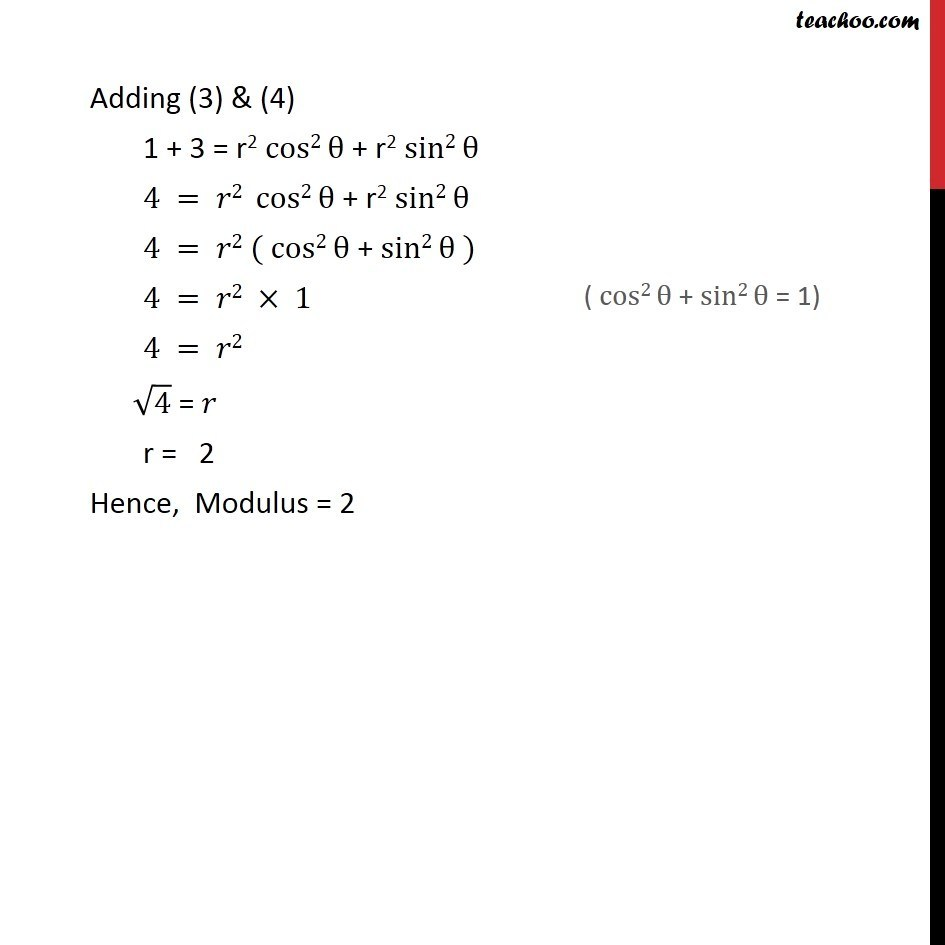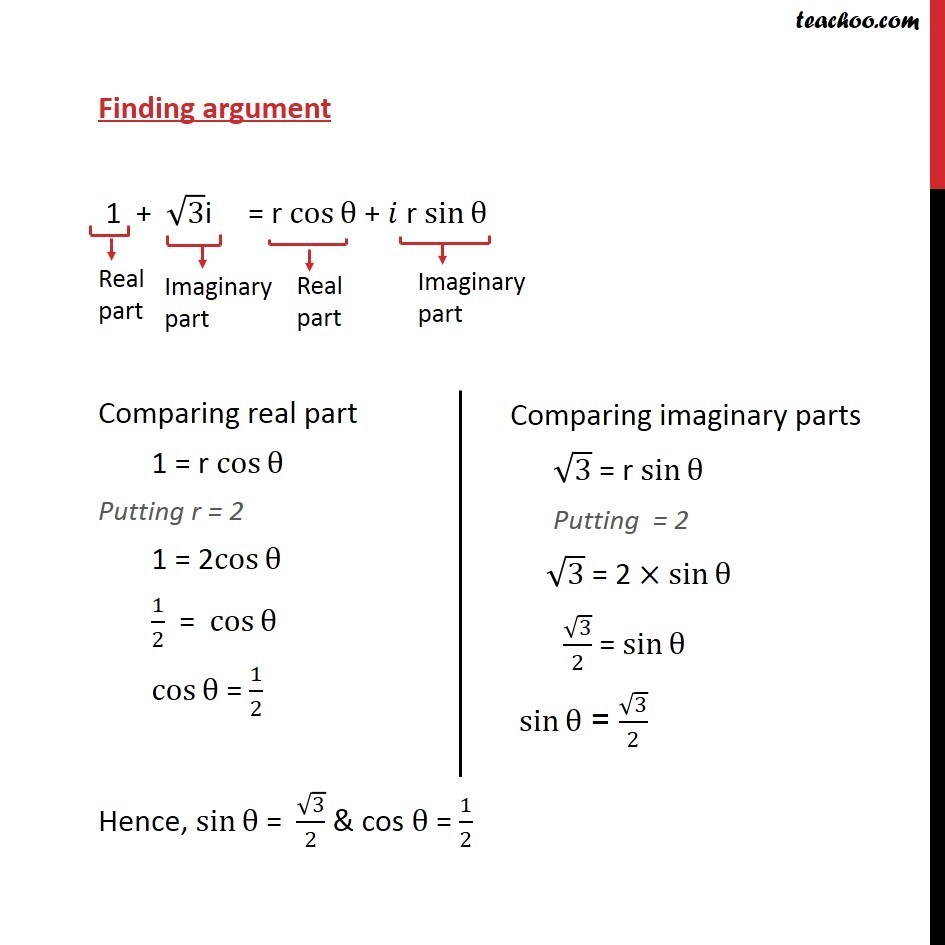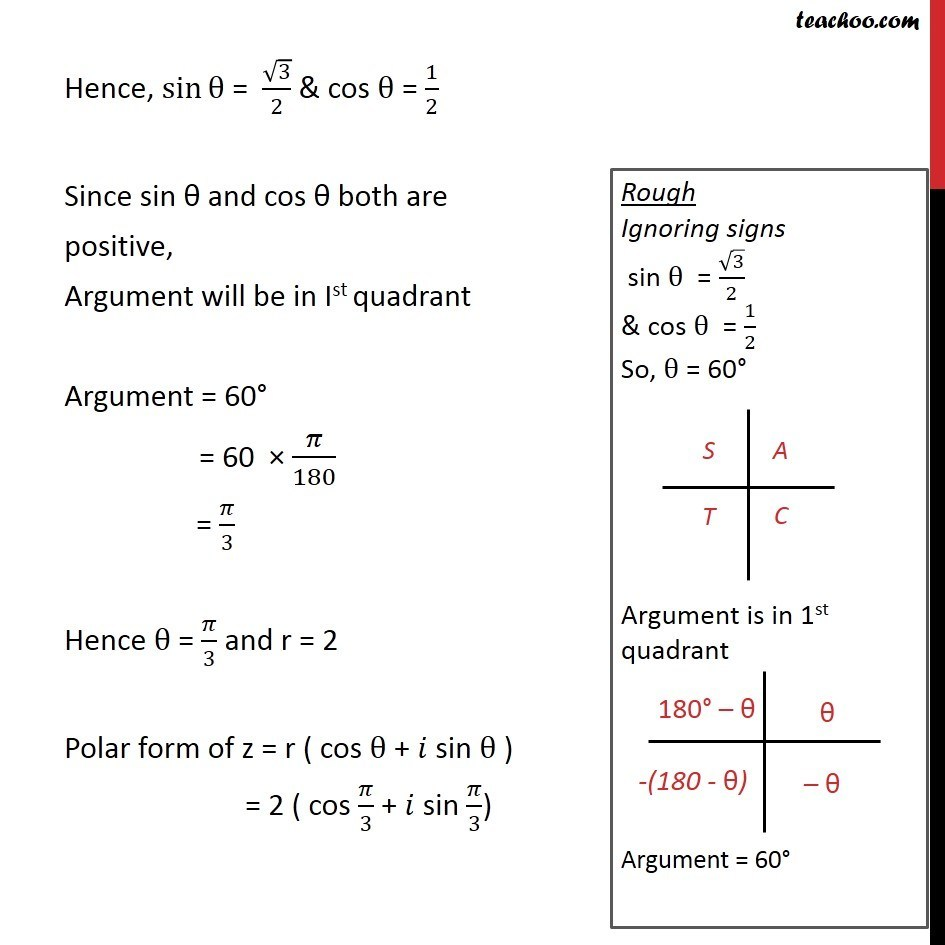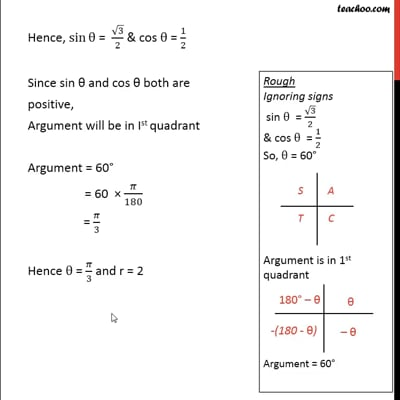This video is only available for Teachoo black users

Introducing your new favourite teacher - Teachoo Black, at only ₹83 per month

### Transcript

Example 7 Convert the given complex number in polar form: 1 + i√3. Given z = 1+ √3i Let polar form be z = r (cos⁡θ + i sin⁡θ) From ( 1 ) & ( 2 ) 1 + √3i = r ( cos⁡θ + i sin⁡θ) 1 + √3i = r〖 cos〗⁡θ + 𝑖 r sin⁡θ Adding (3) & (4) 1 + 3 = r2 cos2⁡θ + r2 sin2⁡θ 4 = 𝑟2 cos2⁡θ + r2 sin2⁡θ 4 = 𝑟2 ( cos2⁡θ + sin2⁡θ ) 4 = 𝑟2 × 1 4 = 𝑟2 √4 = 𝑟 r = 2 Hence, Modulus = 2 Finding argument 1 + √3i = r〖 cos〗⁡θ + 𝑖 r sin⁡θ Comparing real part 1 = r cos⁡θ Putting r = 2 1 = 2cos⁡θ 1/2 = cos⁡θ cos⁡θ = 1/2 Hence, sin⁡θ = √3/2 & cos θ = 1/2 Hence, sin⁡θ = √3/2 & cos θ = 1/2 Since sin θ and cos θ both are positive, Argument will be in Ist quadrant Argument = 60° = 60 × 𝜋/180 = 𝜋/3 Hence θ = 𝜋/3 and r = 2 Polar form of z = r ( cos θ + 𝑖 sin θ ) = 2 ( cos 𝜋/3 + 𝑖 sin 𝜋/3)